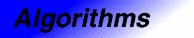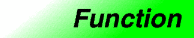set_symmetric_differenceCategory: algorithms Component type: function

Prototype

Set_symmetric_difference is an overloaded name; there are actually two set_symmetric_difference functions.

template <class InputIterator1, class InputIterator2, class OutputIterator>
OutputIterator set_symmetric_difference(InputIterator1 first1,
InputIterator1 last1,
InputIterator2 first2,
InputIterator2 last2,
OutputIterator result);

template <class InputIterator1, class InputIterator2, class OutputIterator,
class StrictWeakOrdering>
OutputIterator set_symmetric_difference(InputIterator1 first1,
InputIterator1 last1,
InputIterator2 first2,
InputIterator2 last2,
OutputIterator result,
StrictWeakOrdering comp);

Description

Set_symmetric_difference constructs a sorted range that is the set symmetric difference of the sorted ranges [first1, last1) and [first2, last2). The return value is the end of the output range.

In the simplest case, set_symmetric_difference performs a set theoretic calculation: it constructs the union of the two sets A - B and B - A, where A and B are the two input ranges. That is, the output range contains a copy of every element that is contained in [first1, last1) but not [first2, last2), and a copy of every element that is contained in [first2, last2) but not [first1, last1). The general case is more complicated, because the input ranges may contain duplicate elements. The generalization is that if a value appears m times in [first1, last1) and n times in [first2, last2) (where m or n may be zero), then it appears |m-n| times in the output range.  Set_symmetric_difference is stable, meaning that the relative order of elements within each input range is preserved.

The two versions of set_symmetric_difference differ in how they define whether one element is less than another. The first version compares objects using operator<, and the second compares objects using a functors comp.

Definition

Defined in the standard header algorithm, and in the nonstandard backward-compatibility header algo.h.

Requirements on types

For the first version:

• InputIterator1 is a model of InputIterator.
• InputIterator2 is a model of InputIterator.
• OutputIterator is a model of OutputIterator.
• InputIterator1 and InputIterator2 have the same value type.
• InputIterator's value type is a model of LessThanComparable.
• The ordering on objects of InputIterator1's value type is a strict weak ordering, as defined in the LessThanComparable requirements.
• InputIterator's value type is convertible to a type in OutputIterator's set of value types.

For the second version:

• InputIterator1 is a model of InputIterator.
• InputIterator2 is a model of InputIterator.
• OutputIterator is a model of OutputIterator.
• StrictWeakOrdering is a model of StrictWeakOrdering.
• InputIterator1 and InputIterator2 have the same value type.
• InputIterator1's value type is convertible to StrictWeakOrdering's argument type.
• InputIterator's value type is convertible to a type in OutputIterator's set of value types.

Preconditions

For the first version:

• [first1, last1) is a valid range.
• [first2, last2) is a valid range.
• [first1, last1) is ordered in ascending order according to operator<. That is, for every pair of iterators i and j in [first1, last1) such that i precedes j, *j < *i is false.
• [first2, last2) is ordered in ascending order according to operator<. That is, for every pair of iterators i and j in [first2, last2) such that i precedes j, *j < *i is false.
• There is enough space to hold all of the elements being copied. More formally, the requirement is that [result, result + n) is a valid range, where n is the number of elements in the symmetric difference of the two input ranges.
• [first1, last1) and [result, result + n) do not overlap.
• [first2, last2) and [result, result + n) do not overlap.

For the second version:

• [first1, last1) is a valid range.
• [first2, last2) is a valid range.
• [first1, last1) is ordered in ascending order according to comp. That is, for every pair of iterators i and j in [first1, last1) such that i precedes j, comp(*j, *i) is false.
• [first2, last2) is ordered in ascending order according to comp. That is, for every pair of iterators i and j in [first2, last2) such that i precedes j, comp(*j, *i) is false.
• There is enough space to hold all of the elements being copied. More formally, the requirement is that [result, result + n) is a valid range, where n is the number of elements in the symmetric difference of the two input ranges.
• [first1, last1) and [result, result + n) do not overlap.
• [first2, last2) and [result, result + n) do not overlap.

Complexity

Linear. Zero comparisons if either [first1, last1) or [first2, last2) is empty, otherwise at most 2 * ((last1 - first1) + (last2 - first2)) - 1 comparisons.

Example

inline bool lt_nocase(char c1, char c2) { return tolower(c1) < tolower(c2); }

int main()
{
int A1[] = {1, 3, 5, 7, 9, 11};
int A2[] = {1, 1, 2, 3, 5, 8, 13};
char A3[] = {'a', 'b', 'b', 'B', 'B', 'f', 'g', 'h', 'H'};
char A4[] = {'A', 'B', 'B', 'C', 'D', 'F', 'F', 'H' };

const int N1 = sizeof(A1) / sizeof(int);
const int N2 = sizeof(A2) / sizeof(int);
const int N3 = sizeof(A3);
const int N4 = sizeof(A4);

cout << "Symmetric difference of A1 and A2: ";
set_symmetric_difference(A1, A1 + N1, A2, A2 + N2,
ostream_iterator<int>(cout, " "));
cout << endl
<< "Symmetric difference of A3 and A4: ";
set_symmetric_difference(A3, A3 + N3, A4, A4 + N4,
ostream_iterator<char>(cout, " "),
lt_nocase);
cout << endl;
}

The output is

Symmetric difference of A1 and A2: 1 2 7 8 9 11 13
Symmetric difference of A3 and A4: B B C D F g H